SYLLABUS  Previous: 6.1 Splitting advection from  Up: 6 LAGRANGIAN METHOD  Next: 6.3 Non-Linear equations with

## 6.2 Cubic-Interpolated Propagation (CIP)

Slide : [ Scheme - Code - Run || VIDEO login]

Introduced a decade ago by Yabe and Aoki , a whole family of schemes have been proposed along the same lines, relying on different interpolations to propagate the solution along the characteristics.

Using a cubic-Hermite polynomial, the discretized function and its first derivative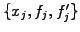is approximated in a continuous manner with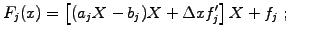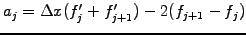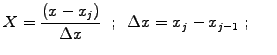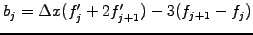Both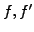satisfy the master advection equation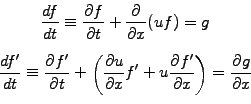(1)

and each is split into an advection (top) and non-advection phase (bottom)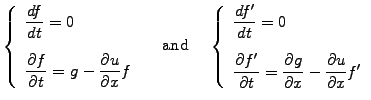(2)

For the advection phase, the solution can be integrated analytically simply by shifting the cubic polynomials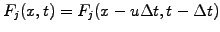along the characteristics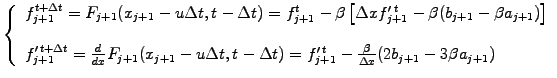(3)

without any restriction on the size or the sign of the Courant-Friedrich-Lewy (CFL) number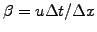(exercise 6.01).

For pedagogical reasons, the scheme implemented in JBONE assumes that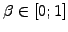so that both quantities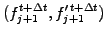can be interpolated from only one piece of the piecewise continuous polynomial, namely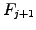defined in the interval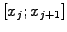. After an initialization where the function is discretized with cubic-Hermite polynomials by sampling on a grid and the derivative are calculated with centered finite differences, the CIP scheme is implemented as

      double alpha=timeStep*diffusCo/(dx*dx);  //These are only constant
double beta =timeStep*velocity/(dx);        //  if the problem and the
int n=f.length-1;                              //  mesh are homogeneous

for (int j=0; j<n; j++) {
a=dx*(df[j]+  df[j+1])-2*(f[j+1]-f[j]);
b=dx*(df[j]+2*df[j+1])-3*(f[j+1]-f[j]);
fp[j+1]=  f[j+1] -beta*(dx*df[j+1]-beta*(b-beta*a));
dfp[j+1]= df[j+1] -beta/dx*(2*b-3*beta*a);
}
a=dx*(df[n]+  df)-2*(f-f[n]);
b=dx*(df[n]+2*df)-3*(f-f[n]);
fp=  f -beta*(dx*df-beta*(b-beta*a));
dfp= df -beta/dx*(2*b-3*beta*a);


The applet below illustrates the high quality of this approach, which combines a low level of dispersion with low damping.

 Numerical experiments: cubic interpolated propagation Change the initial condition to Cosine and reduce the spatial resolution down to 4 and 2 mesh points per wavelength to measure how small both the numerical diffusion and dispersion are in comparison with other schemes. Switch to Cubic--Splines to test an alternative scheme, where cubic splines (1.4.4#eq.1) are used instead of Hermite polynomials to interpolate from any interval in the periodic domain. This allows for arbitrarily large CFL numbers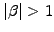as can be tested by choosing TimeStep=21.333 and changing the sign of the Velocity.

Some additional bookkeeping is of course necessary in a code that is intended for: exercise 6.01 deals with exactly this problem and can be implemented in a similar manner as illustrated with the Cubic--Spline scheme.

SYLLABUS  Previous: 6.1 Splitting advection from  Up: 6 LAGRANGIAN METHOD  Next: 6.3 Non-Linear equations with